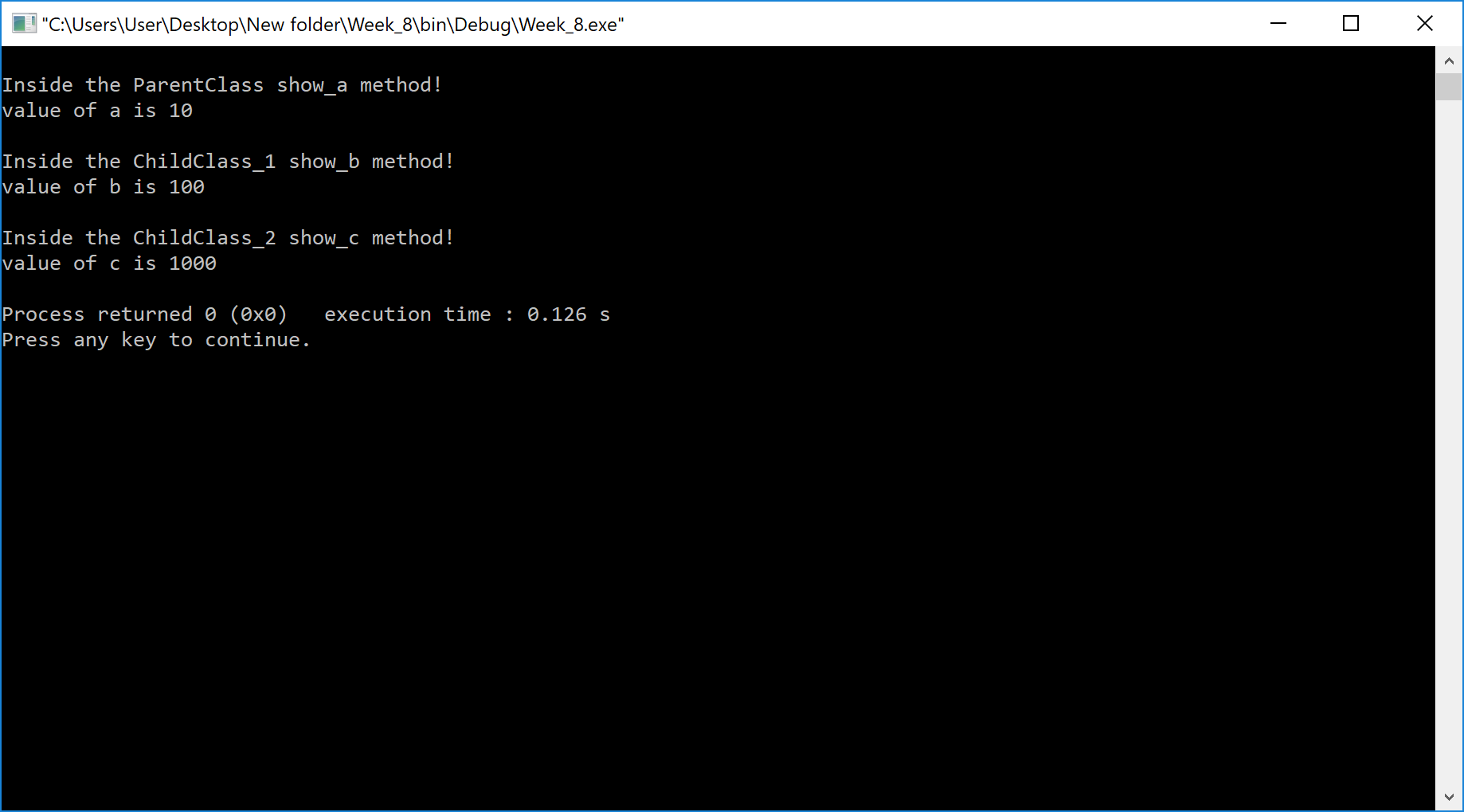The perfect place for easy learning...

×

# Aim

Write a C++ program to create multilevel inheritance. (Hint: Classes A1, A2, A3).

# Solution

In the Multilevel inheritance, the child class derives from a class which already derived from another class. Look at the following example code.

In the following example, we have used public inheritance to implement multilevel inheritance. Here, the ChildClass_2 has derived from ChildClass_1 which was already derived from ParentClass.

# Implementation

Example
``````#include <iostream>
using namespace std;

class  ParentClass{
int a;
public:
ParentClass(){
a = 10;
}
void show_a(){
cout<< endl << "Inside the ParentClass show_a method!" << endl;
cout<< "value of a is " << a << endl;
}

};

class  ChildClass_1:public ParentClass{
int b;
public:
ChildClass_1(){
b = 100;
}
void show_b(){
cout<< endl << "Inside the ChildClass_1 show_b method!" << endl;
cout<< "value of b is " << b << endl;
}

};

class  ChildClass_2:public ChildClass_1{
int c;
public:
ChildClass_2(){
c = 1000;
}
void show_c(){
cout<< endl << "Inside the ChildClass_2 show_c method!" << endl;
cout<< "value of c is " << c << endl;
}

};

int main()
{
ChildClass_2 obj;

obj.show_a();
obj.show_b();
obj.show_c();

return 0;
}
``````

# Result